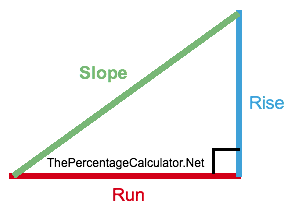Percent Slope Calculator

When driving, have you ever noticed that the warning sign indicating a steep hill has a percentage on it?

This Percent Slope Calculator can calculate that slope percentage!

To get the percent of a slope, simply enter the Rise (height of the hill) and the Run (width of the hill) below and press "Calculate Slope".

Rise:

Run:

Slope:

Above, the percent of the slope, descent, incline, grade, or whatever you want to call it, is rounded to two decimals.

How to Calculate Percent Slope

Here is the Percent Slope formula:

Slope = (Rise/Run)x100

Rise is the height of triangle and Run is the width of a triangle. See illustration below to make it more clear: